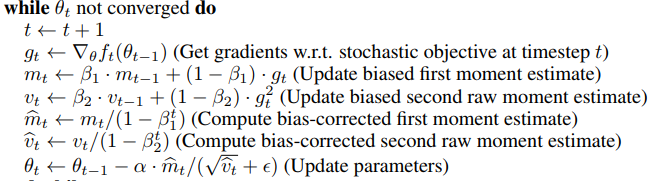# Which optimizer to choose?

fastai implements Adam with the correction mentioned in AdamW and Super-convergence is now the fastest way to train neural nets. Then there is Nadam which incorporated nesterov momentum to Adam.

I am confused about which optimizer does fastai uses actually by default. In the learner default arguments it does say Adam, but I am confused then how fastai uses cyclic momentum. Also, if fastai does use Adam, then can anyone comment on the performance of Nadam.

I am using An overview of gradient descent optimization
algorithms
as a reference reading for optimizers.

We’ll be talking about optimizers in the next lesson2 LikesCan you also discuss a subtle point. In the paper of Adam, when we update the weights we use normalized values of exp_avg and exp_avg_sq (i.e. bias_corrected). But in the pytorch implementation of Adam, they did not use the bias_corrected version when updating the weights.

In the paper, they did say, the bias correction was necessary as exp_avg and exp_avg_sq are initialized as 0, which is True in case of PyTorch also.

The code I am referring to

``````bias_correction1 = 1 - beta1 ** state['step']
bias_correction2 = 1 - beta2 ** state['step']
step_size = group['lr'] * math.sqrt(bias_correction2) / bias_correction1

# PyTorch does not use exp_avg = exp_avg/bias_correction1 in p.data.addcdiv_
``````

I am running some benchmarks to see how much difference that makes.

1 Like

I tried both the above cases, but I found in all my experiments that when I use bias_corrections i.e.

``````exp_avg.div_(bias_correction1)
exp_avg_sq.div_(bias_correction2)
``````

In all the cases, I got much worse scores when using bias_correction. Why is this happening?

The only reason that I can come up with is that it actually makes the learning very slow. As in the weight update equation, the step update size is being divided by 10 when the training begins
(beta1=0.9, beta2=0.999, so
1-beta1 and 1-beta2 = 0.1, 0.0001 and
when we can take them to numerator we get 10, 10000 times exp_grad and exp_grad_sq.
Finally in the update step we take sqrt of 10000 and divide 10/sqrt(10000) = 1/10 of the original gradient update)
As the number of time steps increase the 1/10 factor get’s closer to the original factor, but the cumulative 1/x of the gradient update’s adds on and we have very slow training.

I haven’t tried with cyclic learning for this as of now.

They do.For the first step of algo we use

``````p.grad.data -> to get the gradient
``````

For the next two steps, we calculate m_t and v_t

``````exp_avg.mul_(beta1).add_(1 - beta1, grad)
``````

Then the denominator is calculated to be used for the param update part.

``````denom = exp_avg_sq.sqrt().add_(group['eps'])
``````

In the paper, we use the bias corrected version of exp_avg_sq to caculate the denominator, while in pytorch they do not use the bias_corrected one.

So the pytorch implementation skips the calculation of m_t_hat and v_t_hat.

Then the biases are calculated for setting the adaptive learning rate of each param.

``````bias_correction1 = 1 - beta1 ** state['step']
bias_correction2 = 1 - beta2 ** state['step']
``````

But we never use these to change the values of m_t and v_t.

I got my mistake.

When we simplify the equations for the param update we do get `alpha*srqt(beta2)/beta1` which is the step_size and we do not have to do the m_t_hat and y_t_hat steps.

Spent complete evening, for this and now I understand why my experiments were failing. But now I can write any optimizer function. Thanks, @jeremy for helping along the way.

2 Likes